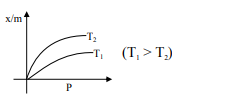# Select the graph that correctly describes the adsorption isotherms at two temperatures`
Question:

Select the graph that correctly describes the adsorption isotherms at two temperatures $T_{1}$ and $T_{2}$ $\left(\mathrm{T}_{1}>\mathrm{T}_{2}\right)$ for a gas :

$(\mathrm{x}-$ mass of the gas adsorbed ; $\mathrm{m}-$ mass of adsorbent; P-pressure)

1.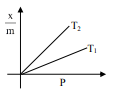2.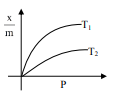3.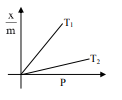4.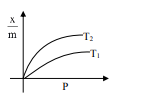Correct Option: , 4

Solution:

$\frac{\mathrm{x}}{\mathrm{m}} \alpha \mathrm{P}^{1 / \mathrm{n}}\left(0<\frac{1}{\mathrm{n}}<1\right)$

On Increasing temperature $\frac{x}{m}$ decreases.

$\because$ adsorption is generally exothermic